﻿ Resolution and Brightness Enhancement of Images by Using Singular Value Decomposition
Journal of Telecommunications System & Management
All submissions of the EM system will be redirected to Online Manuscript Submission System. Authors are requested to submit articles directly to Online Manuscript Submission System of respective journal.
• Research Article
• J Telecommun Syst Manage 2016, Vol 5(2): 138
• DOI: 10.4172/2167-0919.1000138

# Resolution and Brightness Enhancement of Images by Using Singular Value Decomposition

Subha S1*, Jesudass I1 and Thanushkodi K2
1Department of ECE, KLN college of Engineering, Madurai, India
2Akshaya college of Engineering and Technology, Coimbatore, India
*Corresponding Author: Subha S, Department of ECE, KLN college of Engineering, Madurai, India, Tel: 91-452-6562171, Email: [email protected]

Received Date: Jan 24, 2016 / Accepted Date: Feb 23, 2016 / Published Date: Mar 05, 2016

### Abstract

Image denoising plays an important role in digital image processing. There are many ways to denoise an image, which including gradient-based, sparse representation-based, non-local self-similarity-based, Gradient Histogram Preservative (GHP) algorithm. By using GHP algorithms noise can be removed, but it degrads the visual quality of an original image and also execution time to generate the denoised image is large. To avoid this problem, in this paper, we propose a Discrete Wavelet Transform (DWT), Stationary wavelet transform (SWT) and Singular Value Decomposition (SVD) method. This method is developed to enhance brightness and resolution while removing noise and execution time should be reduced. Our experimental results demonstrate that the proposed Discrete Wavelet Transform (DWT), Stationary wavelet transform (SWT) and Singular Value Decomposition (SVD) method can well preserve the texture appearance in the denoised images and improve the resolution of denoised image and execution time should be reduced.

Keywords: Image denoising; Wavelet transform; SVD; GHP algorithm

#### Introduction

IMAGE denoising is a classical and flourishing research topic in image processing. The demand for image denoising approaches comes from the fact that during the process that a digital image is captured, quantized, recorded and transmitted. A great deal of image denoising algorithms have been proposed originating from various subjects ranging from probability and statistics to differential equations, from spatial-domain filtering to transform-domain spectrum manipulation. Generally, image denoising is an important topic of image restoration , which aims at reconstructing an image with a certain degree of degradation. A generally degradation model of an image can be described as follows:

v(x) = u(x) + n(x)

Where x represents the two-dimensional location of a pixel, u denotes the original image we want to reconstruct and v is the noisy observation of the image and n denotes the noise, which can be arbitrary digital noise that could occur, such as pulsing noise, salt-and– pepper noise as well as Gaussian valued noise. Usually, when we talk about image denoising, we refer the noise as additive white Gaussian noise (AWGN). The goal of image denoising is to find out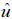, which is the estimate of original image u(x) from its noisy observation v(x). Several criterions exist to evaluate the denoising performance of different denoising algorithms. Among which the PSNR is probably the most widely used one. A higher PSNR indicates a better performance of the algorithm. PSNR is short for Peak Signal to Noise Ratio. Generally in signal processing, signal-to-noise-ratio is used to represent the proportion of power between the signal and the noise.

Image resolution enhancement in the wavelet domain is a relatively new research addition, and recently, many new algorithms and others have estimated at the unknown details of wavelet coefficients in an effort to improve the sharpness of reconstructed images . The 1-D DWT can be extended to 2-D transform using separable wavelet filters. With separable filters, applying a 1-D transform to all the rows of the input and then repeating on all of the columns can compute the 2-D transform. When one-level 2-D DWT is applied to an image, four transform coefficient sets are created. The four sets are LL, HL, LH, and HH, where the first letter corresponds to applying either a low pass or high pass filter to the rows, and the second letter refers to the filter applied to the columns (Figure 1).

#### Related Work

Image denoising methods can be grouped into two categories. They are Model-based method and Learning based method. Aim of the image denoising is to get the clean image from noisy category image. Most denoising methods reconstruct the clean image by exploiting some image and noise prior models, and belong to the first Learning-based methods attempt to learn a mapping function from the noisy image to the clean image, and have been receiving considerable research interests. Studies on natural image priors aim to find suitable models to describe the characteristics or statistics (e.g., distribution) of images in some domain.

#### Existing Denoising Algorithms

A brief overview

Plenty of denoising algorithms are proposed. Among these algorithms, some perform spatial convolution locally, such as the Gaussian smooth model, some apply spatial convolution in a global range, such as non-local means ; some achieve denoising by anisotropic filtering based on the texture and edge information of the image. Some exploit the prior information of the image in both Euclidean space as well as the Intensity space. Also there are some algorithms are available to analyze the denoising problem in frequency domain, such as BM3D, as well as some approaches using wavelet thresholding. Another class to discuss is the optimization-based algorithms, which typically achieve denoising by calculus of variations, such as Total Variation Minimization.

Isotropic filtering

By Riesz theorem, isotropic filtering of image is equivalent to a convolution of the image by a linear symmetric kernel. The most famous isotropic filter is the Gaussian kernel: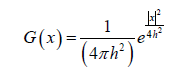Where, h is the standard deviation and the estimated image is then represented as the convolution of the noisy observation and the Gaussian kernel: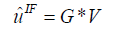In practice the filtering range is the rectangle neighborhood around the current pixel. Though Gaussian isotropic filtering is effective to remove slightly contaminated images and simple to implement.

SUSAN filtering

SUSAN filtering  is a kind of neighborhood filtering which shares the same idea as Bilateral filtering . Instead of consider only the spatial neighborhood which is close to the current pixel spatially, SUSAN filter weights pixels both by the Euclidean distance and the Intensity-space distance, i.e.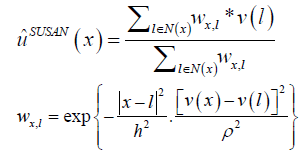By exploiting the inherent intensity structure of the image, The SUSAN filter can preserves sharp edges and textures when removing the noise, which is also an important property of anisotropic filter.

Another advantage of SUSAN filter (Bilateral filter) is that unlike the anisotropic filtering, it is a non-iterative edge- preserving denoising algorithm, which makes it widely used, even adopted by the well-known Adobe Photoshop.

The noisy observation y of an unknown clean image x is usually modeled as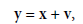(1)

Where, v is the additive white Gaussian noise (AWGN) with zero mean and standard deviation σ. The goal of image denoising is to estimate the desired image x from y. One popular approach to image denoising is the variational method, in which the denoised image is obtained by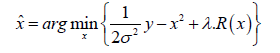(2)

Where R(x) denotes some regularization term and λ is a positive constant. The specific form of R(x) depends on the employed image priors. One common problem of image denoising methods is that the image fine scale details such as texture structures will be over-smoothed. An over-smoothed image will have much weaker gradients than the original image. Intuitively, a good estimation of x without smoothing too much the textures should have a similar gradient distribution to that of x. With this motivation, we propose a gradient histogram preservation (GHP) model  for texture enhanced image denoising. Suppose that we have an estimation of the gradient histogram of x, denote by hr. In order to make the gradient histogram of denoised image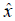nearly the same as the reference histogram hr, we propose the following GHP based image denoising model: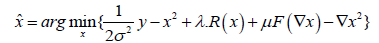(3)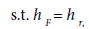Where F denotes an odd function which is monotonically non-descending, hF denotes the histogram of the transformed gradient image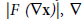denotes the gradient operator, and μ is a positive constant.

The existing GHP model can be formulated as: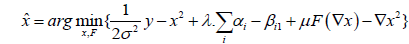(4)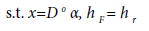If the histogram regularization parameter μ is high, the function F (∇x) will be close to ∇x. Since the histogram hF of |F (∇x)| is required to be the same as hr, the histogram of ∇x will be similar to hr, leading to the desired gradient histogram preserved image denoising.

Iterative histogram specification algorithm

The proposed GHP model in Eq. (4) can be solved by using the variable splitting (VS) method, which has been widely adopted in image restoration [4,7-9]. By introducing a variable g = F (∇x), we adopt an alternating minimization strategy to update x and g alternatively. Given g = F (∇x), we update x (i.e., α) by solving the following sub-problem: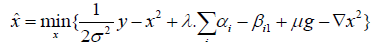(5)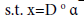We use the method in  to construct the dictionary D adaptively. Based on the current estimation of image x, we cluster its patches into K clusters, and for each cluster, a PCA dictionary is learned. Although in Eq. (5) the l1-norm regularization is imposed on ||αii||1 rather than ||αi||1, by introducing a new variable ϑii −βi, we can use the iterative shrinkage/thresholding method  to update ϑi and then update αiii. This strategy is also used in  to solve the problem with this regularization term, and thus here we omit the detailed deduction process. To get the solution to the sub-problem in Eq. (5), we first use a gradient descent method to update x: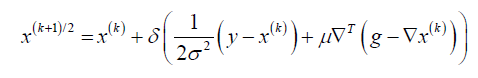(6)

Where δ is a pre-specified constant. Then, the coding coefficients αi are updated by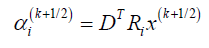(7)

Once the estimate of image x is given, we can update F by solving the following sub-problem: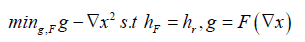(8)

Considering the equality constraint g = F (∇x), we can substitute ‘g’ in || g −∇x ||2with F (∇x), and the sub-problem becomes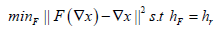(9)

Existing algorithm

1. Initialize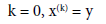2. Iterate on k = 0,1,2,…,J

3. Update g:

g =F(∇x)

4. Update x: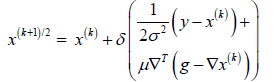5. Update the coding coefficients of each patch: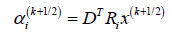6. Update the nonlocal mean of coding vector: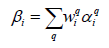7. Update α: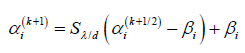8. Update x: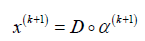9. kk + 1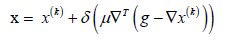#### Proposed Method

The proposed image resolution enhancement technique based on high frequency sub-band images obtained by discrete wavelet transforms (DWT) of the interpolated input image. The edges are enhanced by introducing an intermediate stage by using stationary wavelet transform (SWT).

DWT is applied in order to decompose an input image into different sub-bands. The high frequency sub-bands of DWT are being modified by using high frequency sub-band obtained through SWT. Then all these sub-bands are combined to generate a new high resolution image by using inverse DWT (IDWT). To increase the brightness of image, use SVD (singular value decomposition) and DWT at the output of resolution enhanced image. In this method of resolution enhancement DWT using Daubechies (Db9/7) wavelet as mother wavelet function and bicubic interpolation as interpolation technique.

Block diagram of proposed technique is shown in Figure 2. It consists of two steps. In first step, resolution enhancement uses the DWT and SWT, and in second step brightness enhanced by using DWT and SVD.

Resolution enhancement

Resolution is an important feature in satellite imaging, which makes the resolution enhancement of such images, will directly affect the performance of the system using these images as input. The main loss of an image after being resolution enhanced by applying interpolation is on its high frequency components, which is due to the smoothing caused by interpolation. Hence, in order to increase the quality of the enhanced image, preserving the edges is essential. DWT has been employed in order to preserve the high-frequency components of the image .

Down sampling in each sub-band of DWT causes information loss in the respective sub-band [13-15]. So this can be minimized by using SWT along with DWT. In the proposed method, input image is interpolated with factor 2, and then DWT applied to these interpolated images. DWT separates these images into different sub-band images, namely, LL, LH, HL, and HH. High-frequency sub-bands contain the high frequency component of the image.

The interpolated high frequency sub-band and the SWT sub-band are added with each other. The new corrected high frequency sub-band can be interpolated with factor α/2 for higher enlargement. The low-frequency sub-band image, which contains less information than the original input image, so we are using this input image through the interpolation process. Using input image instead of low frequency sub-band image increases the quality of enhanced image.

The interpolated input image and the high frequency sub-bands are combined by using inverse DWT (IDWT), to produce the high resolution image.

Brightness enhancement

This is the second step of the proposed technique. At first apply DWT to the output of first module, and then calculate the singular value of LL sub-band image using SVD. Then applying parallelly the histogram equalization at the high resolution image (i.e. the output obtained from the first module). Histogram equalization is a process of automatically determining transformation function which produces an output image with a uniform histogram.

Applying the DWT to the histogram equalized image, it produces different sub-band i.e. LL, LH, HL&HH. Then calculate the singular value of LL band using SVD. The singular value matrix obtained by SVD contains the illumination information. So we have to change this matrix to change the brightness of the image. Any changes made to this matrix will not affect the other attributes of the image. Combining the two SVD output will produce new LL band and is given by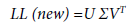(10)

Where U is an m×m real or complex unitary matrix, Σ is an m×n rectangular diagonal matrix which contains the illumination information of an image, and VT (the conjugate transpose of V) is an n×n real or complex unitary matrix.

Then applying the inverse DWT. IDWT has been applied to combine all these images to generate the final brightness enhanced image.

Proposed method

Described in Figure 2.

#### Experimental Results

To verify the performance of the proposed Discrete (DWT), Stationary Wavelet Transform (SWT) and Singular Value Decomposition (SVD) method, we apply it test images with various texture structures. All the test images are gray-scale images with gray level ranging from 0 to 255. Finally, experiments are conducted to validate its performance in comparison with the state-of-the-art denoising algorithms.

Here peak signal-to-noise ratio (PSNR) has been used to obtain the quantitative results for comparison.

PSNR=10log10 (R2/MSE) (11)

Where R is the maximum fluctuation in the input image.

#### Simulation Result

Mentioned in Figures 3-8 and Tables 1 and 2.

Iterations Sigma = 20 Sigma = 30
Base paper result GHP algorithm result SVD  Algorithm result Base paper result GHP algorithm result SVD Algorithm result
1 30.59 27.94 28.35 28.47 29.04 29.89
2 27.9 28.7 29.41 25.87 27.62 28.46
3 28.15 29.28 29.93 26.34 27.12 28.33
4 26.59 29.45 30.06 24.46 25.41 27.01
5 30.54 30.36 30.55 28.61 28.53 28.99
6 28.39 29.97 31.01 26.12 28.71 29.31
7 30.07 31.01 30.27 28.15 29.24 29.87
8 31.27 30.66 30.89 29.23 29.12 30.08
9 27.31 30.71 31.26 25.21 28.63 29.98
10 30.83 30.86 31.69 28.85 29.45 30.57
Average 29.16 29.89 30.35 27.13 28.18 29.25

Table 1: PSNR value comparison between the base paper and experimental result.

Image file format Iteration 1 PSNR value Iteration 2 PSNR value Iteration 3 PSNR value Iteration 4 PSNR value Iteration 5 PSNR value Computational time
TIF 28.35 29.41 29.93 30.06 30.55 Approximately 30min
BMP 29.31 29.79 30.23 30.76 31.09 Approximately 35min
JPG 28.56 28.01 28.21 30.66 29.76 Approximately 30min

Table 2: PSNR value of de-noised images for zero mean and Standard Deviation=20.

#### Conclusion

In this paper, we presented a Discrete (DWT), Stationary Wavelet Transform (SWT) and Singular Value Decomposition (SVD) method for texture-enhanced image denoising. An efficient proposed method and algorithm was developed. This proposed method and algorithm achieves promising results in enhancing the texture structure while removing random noise. The experimental results demonstrated the effectiveness of method and algorithm. This method leads to PSNR measures to the state-of-the-art denoising method. However, it leads to more natural and visually pleasant denoising results by better preserving the image texture areas. Most of the state-of-theart denoising algorithms are based on the local sparsity and nonlocal self-similarity priors of natural images. Limitations of this method are that it cannot be directly applied to non-additive noise removal. The computational time is approximately 30 min to generate denoised image.

#### References

Citation: Subha S, Jesudass I, Thanushkodi K (2016) Resolution and Brightness Enhancement of Images by Using Singular Value Decomposition. J Telecommun Syst Manage 5: 138. Doi: 10.4172/2167-0919.1000138

Copyright: ©2016 Subha S. This is an open-access article distributed under the terms of the Creative Commons Attribution License, which permits unrestricted use, distribution, and reproduction in any medium, provided the original author and source are credited.

Select your language of interest to view the total content in your interested language

##### Recommended Journals
Viewmore
###### Article Usage
• Total views: 11639
• [From(publication date): 9-2016 - Nov 21, 2019]
• Breakdown by view type
• HTML page views: 11472Can't read the image? click here to refresh